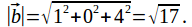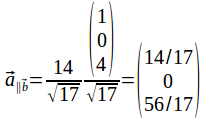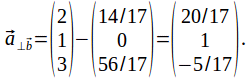## The Projection of a Vector Onto Another Vector

If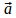and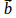are two vectors then we can expressas the sum of two vectors. One of these vectors is that component ofparallel toand the other part is the compe=onent ofperpendicular to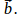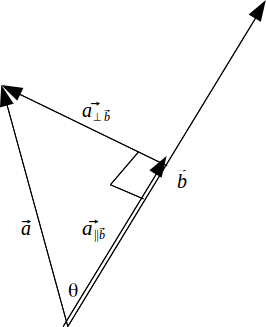If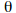is the angle betweenandthen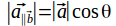and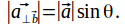Since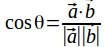we have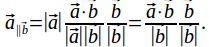Write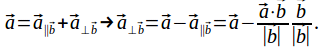Example: If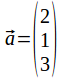and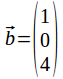then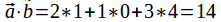and